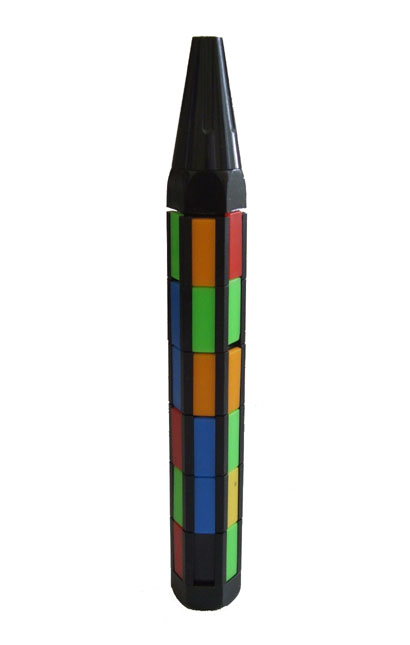### Problem Statement

PenPuzzle is a variation on the famous Rubik's cube. Imagine a polygonal cylinder with k sides, where each side is divided into m slots (for a total of k*m slots). All slots are of the same height, so the slots form several levels (the lowermost slots on each side form one level, the slots directly above those form another level, and so on). Each slot contains a single colored plate. There are k different colors, and m plates of each color. The goal of the game is to arrange the plates such that each side contains only plates of the same color. The following picture shows a 6-sided puzzle with 6 levels on each side:You can use the following two actions to solve the puzzle. First, any level can be rotated around the axis of the cylinder in any direction. A single rotation will move each plate on a level to the slot on the next side at the same level. For example, if we take the puzzle from the picture and rotate level 2 (the second from the bottom) in a clockwise direction, the blue plate will move to the green plate's original position, and the yellow plate will take the blue plate's place. Each such rotation takes 1 second. A full turn around the example puzzle would therefore take 6 seconds.

The second allowed action is shifting plates within a single side. If there's an empty slot directly adjacent to a plate on the same side, the plate can be moved into that slot. For example, the bottommost slot on the middle side of the example puzzle (between the red and green plates) is empty, so the blue plate from the second level can be moved to the first level (leaving its original slot on the second level empty). This movement also takes 1 second. Please note that neither the red nor green plate on the bottommost level can be moved to the empty slot because the puzzle is only constructed to allow plates to be shifted within the same side. If we were to rotate the first level counterclockwise, the empty slot would replace the green plate, the red plate would replace the empty slot, etc.

You will be given a String[] puzzle representing the initial state of the puzzle. Each element of puzzle represents one level of the puzzle, with the first k uppercase letters representing the k different colors. The i-th character of each element of puzzle corresponds to the i-th side. You must remove any one plate from the puzzle to create an empty slot and make shifting plates possible. Find the plate which will allow you to solve the puzzle in the minimal time and return this time.

### Definition

 Class: PenPuzzle Method: solve Parameters: String[] Returns: int Method signature: int solve(String[] puzzle) (be sure your method is public)

### Constraints

-puzzle will contain between 2 and 3 elements, inclusive.
-All elements of puzzle will contain the same number of characters.
-Each element of puzzle will contain between 3 and 4 characters, inclusive.
-Each element of puzzle will contain only the first k uppercase letters, where k is the length of any element of puzzle. All elements of puzzle will contain the same total number of appearances of each of the first k uppercase letters.

### Examples

0)

 `{"ABC", "ABC"}`
`Returns: 0`
 This puzzle is already solved. You can remove any plate and it still will be solved.
1)

 `{"ABC", "BCA"}`
`Returns: 1`
 One rotation solves this puzzle.
2)

 `{"ABA", "BCC"}`
`Returns: 2`
 The initial state of the puzzle is ```ABA BCC ``` Remove the second 'A' plate to get to the following configuration ('_' stands for an empty slot): ```AB_ BCC ``` Shift the second 'C' to the upper level: ```ABC BC_ ``` And rotate the upper row to solve the puzzle: ```BCA BC_ ```
3)

 `{"CBBC", "DCAB", "ADAD"}`
`Returns: 13`

#### Problem url:

http://www.topcoder.com/stat?c=problem_statement&pm=6667

#### Problem stats url:

http://www.topcoder.com/tc?module=ProblemDetail&rd=10093&pm=6667

Olexiy

#### Testers:

PabloGilberto , brett1479 , vorthys

Graph Theory